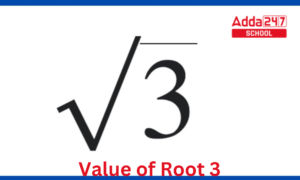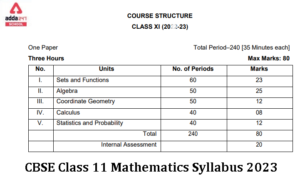Online Tution   »   Important Question   »   What is the Square Root of...

# What is the Square Root of 4?

## What is the Square Root of 4?

Square Root of 4: Finding the Square root of a number is finding a number that when multiplied by itself gives the number whose square root we are looking for. In simpler words we can say that square root is the reverse process of doing a square of a number. So, here to find the square root of 4, we need to find the number whose square is 4.

## What’s the Square Root of 4?

According to the mathematical operations, Square root of a number x is a number y such that y2 = x.  Or we can say that, a number y whose square (the product of the number by itself, or we can write it as y × y or -y × -y) is x.

The Square root of any number is represented by the symbol. Any number which will be placed under this sign () will automatically be reduced to its square root. For example, if x is a number and it is written as, then we have find a number y, whose square is x, i.e. y2 = x. Then ±y will be the square root of x, and hence the required value.

Compound Interest Formula, Definition, Calculator, Questions and Examples

## How to find a square root of 4?

To find the square root of a number, we find a number whose square is the given number. Sometimes, the number is small and the square root can be found out easily, but sometimes there are big numbers and we cannot find the square root just by looking at it. For that, we find the factors of the given number, whose square root we need to find and then, we find all the factors of the number. Then, we arrange the numbers in such a way that they are distributed in two equal sets of factors. Then each set of numbers represent the square root of given number.

For example, We are given a number A, and the factors of A are x × y × z × z × y × x. Then, we see here that we can distribute the factors as two sets of x × y × z, and hence the square root of A is the number obtained by the product of x, y, and z.

Also the negative set of the product of  x, y, and will also be the square root of A. Therefore, every square has two roots, one positive and one negative.

Therefore, (x × y × z) and -(x × y × z) are the two square roots of A.

Perimeter Of Square And Its Formula

## What is the square root of 4 answer?

As we have seen above that to find the square root of a number, we find a number whose square is the given number. And in order to do so, we need to find the factors of the given number, (here the number is 4) and distribute them equally. So, finding the factors of 4,

4 = 2 × 2

So, here we have two sets of 2.

Therefore, the square root of is 2.

Also, we know that can be written as

4 = -2 × -2

So, here we have two sets of -2.

Therefore, -2 is also the square root of 4.

Hence, the square root of 4,

 √(4) = ± 2

CBSE Class 12 Maths Sample Paper 2022-23 With Solutions

## What is the Square Root of 4?: FAQs

How do you find 4 roots?

A number’s fourth root is the same as its square root’s fourth root. Symbolically, the fourth root is also shown by multiplying the radical by 4.

How do you simplify square root of 4?

To simplify the square root of 4, to find the square root of a number, we find a number whose square is the given number. And in order to do so, we need to find the factors of the given number, (here the number is 4) and distribute them equally, i.e. 4 = 2 × 2. Hence square root of 4 is 2.

How do I find square root?

Finding the Square root of a number is finding a number that when multiplied by itself gives the number whose square root we are looking for. In simpler words we can say that square root is the reverse process of doing a square of a number.

What is the Value of the Square Root of 4?

The value of square root of 4 is 2.

Why is √4 a irrational number?

Since 2 is a whole number and all whole numbers are rational, the provided number, 4, equals 2. Additionally, it can be written as 2 1, indicating that it is a rational number. √4 is not an irrational number as a result.

Sharing is caring!

## FAQs

### How do you find 4 roots?

A number's fourth root is the same as its square root's fourth root. Symbolically, the fourth root is also shown by multiplying the radical by 4.

### How do you simplify square root of 4?

To simplify the square root of 4, to find the square root of a number, we find a number whose square is the given number. And in order to do so, we need to find the factors of the given number, (here the number is 4) and distribute them equally, i.e. 4 = 2 × 2. Hence square root of 4 is 2.

### How do I find square root?

Finding the Square root of a number is finding a number that when multiplied by itself gives the number whose square root we are looking for. In simpler words we can say that square root is the reverse process of doing a square of a number.

### What is the Value of the Square Root of 4?

The value of square root of 4 is 2.

### Why is √ 4 a irrational number?

Since 2 is a whole number and all whole numbers are rational, the provided number, 4, equals 2. Additionally, it can be written as 2 1, indicating that it is a rational number. √4 is not an irrational number as a result.

Thank You, Your details have been submitted we will get back to you.

### TOPICS:

•Class 12 Maths Important Question With S...
•CBSE Maths Sample Paper Class 12 2023 wi...
•What is the Value of Root 3?
•CBSE Class 11 Maths Syllabus 2022-23 PDF...
•CBSE Class 10 Maths Syllabus 2022-23 PDF...
•CBSE Important Questions for Class 10 Ma...